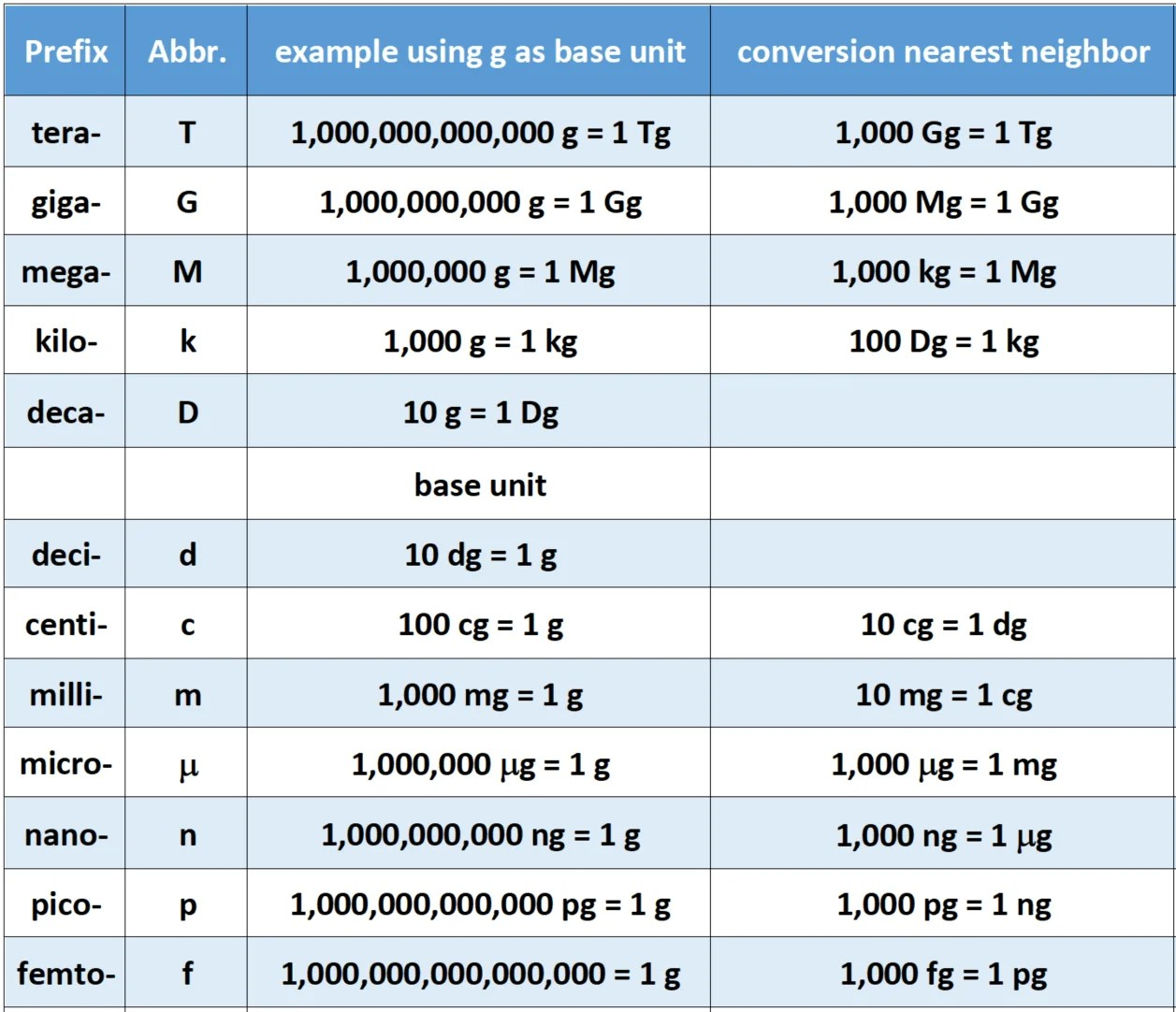## [Sample Lesson] ▣ Chapter 1 - Introduction to Physics

1. Physics and the Laws of Nature

• Physics: the study of the fundamental laws of nature
• these laws can be expressed as mathematical equations
• much complexity can arise from relatively simple laws

2. Units of Length, Mass, and Time

• SI units of length (L), mass (M), time (T):
• Length: the meter
• Was: one ten-millionth of the distance from the North Pole to the equator
• Now: the distance traveled by light in a vacuum in 1/299,792,458 of a second
• Mass: the kilogram
• One kilogram is the mass of a particular platinum-iridium cylinder kept at the International Bureau of Weights and Standards, Sèvres, France.
• Time: the second
• One second is the time for radiation from a cesium-133 atom to complete 9,192,631,770 oscillation cycles.3. Dimensional Analysis

• Any valid physical formula must be dimensionally consistent
• Each term must have the same dimensions
• Distance = velocity × time
• Velocity = acceleration × time
• Energy = mass × (velocity)²

4. Significant Figures

• accuracy of measurements is limited
• significant figures: the number of digits in a quantity that are known with certainty
• number of significant figures after multiplication or division is the number of significant figures in the least-known quantity
• Example:
• A tortoise travels at 2.51 cm/s for 12.23 s. How far does the tortoise go?
• Answer: 2.51 cm/s × 12.23 s = 30.7 cm (three significant figures)

❖ Scientific Notation

• Leading or trailing zeroes can make it hard to determine number of significant figures: 2500, 0.000036
• Each of these has two significant figures
• Scientific notation writes these as a number from 1-10 multiplied by a power of 10, making the number of significant figures much clearer:
• 2500 = 2.5 × 10³ If we write 2.50×10³, it has three significant figures 0.000036 = 3.6 x 10⁻⁵
• Round-off error:
• The last digit in a calculated number may vary depending on how it is calculated, due to rounding off of insignificant digits
• Example:
• \$2.21 + 8% tax = \$2.3868, rounds to \$2.39
• \$1.35 + 8% tax = \$1.458, rounds to \$1.49
• Sum:
• \$2.39 + \$1.49 = \$3.88
• \$2.21 + \$1.35 = \$3.56
• \$3.56 + 8% tax = \$3.84

5. Converting Units

• Converting feet to meters: 316ft = ? m
• 1 m = 3.281 ft (this is a conversion factor)
• Or: 1 = 1 m / 3.281 ft
• 316 ft × (1 m / 3.281 ft) = 96.3 m
• Note that the units cancel properly – this is the key to using the conversion factor correctly.